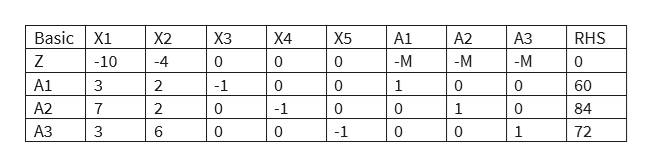# Consider the following LP model in standard form, with a row for the objective function Z.a) Put it into Canonical form ( or Simplex Tableau form) with basic variables X1, X2 , and X3.b) Determine the association BFS (Basic Feasible Solution) and the new formula for the objective function ZMinimize  10X1 + 4X2Sujbject to 3X1 + 2X2 - X3           = 607X1 + 2X2       - X4      = 843X1 + 6X2           -X5 = 72  X1,  X2,  X3 ,  X4 ,  X5 >= 0

Question
15 views

Consider the following LP model in standard form, with a row for the objective function Z.

a) Put it into Canonical form ( or Simplex Tableau form) with basic variables X1, X2 , and X3.

b) Determine the association BFS (Basic Feasible Solution) and the new formula for the objective function Z

Minimize  10X1 + 4X2

Sujbject to

3X1 + 2X2 - X3           = 60

7X1 + 2X2       - X4      = 84

3X1 + 6X2           -X5 = 72

X1,  X2,  X3 ,  X4 ,  X5 >= 0

check_circle

Step 1

Tableau Form

The variables x3, x4 and x5 are having negative coefficients and hence they will get a negative value if they are made the basic variables, leading to infeasible solution. Hence, we cannot have x1, x2 and x3 as the basic variables in the starting tableau (they may enter the basic set in the subsequent steps). So we need to use artificial variables in the three equations to get a starting basic feasible solution and proceed with the Big-M method or 2 phase method for solving the LP. Using the artificial variables A1, A2 and A3 for the three equations and then including them in the objective function with large positive coefficient M (to penalize these artificial variables) we get the following format:

Minimize z = 10x1 + 4x2 +MA1 + MA2 + MA3

subject to

3X1 + 2X2 - X3 + A1          = 60

7X1 + 2X2       - X4 + A2     = 84

3X1 + 6X2           -X5 + A3 = 72

X1,  X2,  X3 ,  X4 ,  X5,  A1, A2, A3 >= 0

Step 2

Artificial Variables

Hence the simplex tableau form is show below:

But the basic variables A1, A2 and A3 need to have 0 values in the z row as they are part of the starting basic feasible solution. Hence we need to substitute out the variables A1, A2 and A3 in the z-equation.

z = 10 x1 + 4x2 + MA1 + MA2 + MA3

A1 = 60 – 3x1 – 2x2 +x3       (from constraint 1)

A2  = 84 – 7x1 – 2x2 +x4      (from constraint 2)

A3 = 72 – 3x1 – 6x2 + x5      (from constraint 3)help_outlineImage TranscriptioncloseBasic X1 RHS X2 ХЗ Х4 X5 A1 A2 АЗ Z -M -M -10 4 0 0 10 -M A1 3 2 -1 0 0 1 0 60 A2 7 2 0 -1 1 84 АЗ 3 6 0 0 -1 0 0 1 72 fullscreen
Step 3

Modified z-equation

Therefore using these values of A1, A2 and A3 in z-equation we get

z = 10x1 + 4x2 +M(60-3x1-2x2+x3)+M(84-7x1-2x2+x4)+M(72-3x1-6x2+x5)

= 10x1 +4x2+60M-3Mx1-2Mx2+M...

### Want to see the full answer?

See Solution

#### Want to see this answer and more?

Solutions are written by subject experts who are available 24/7. Questions are typically answered within 1 hour.*

See Solution
*Response times may vary by subject and question.
Tagged in